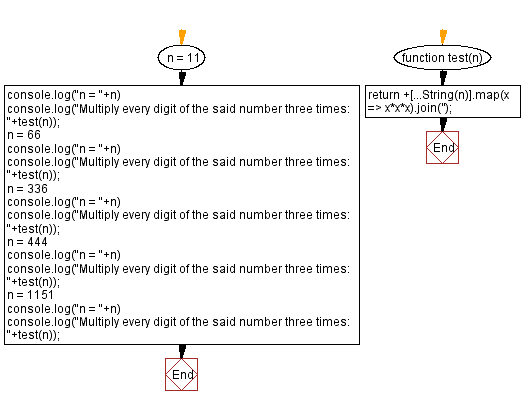# JavaScript: Multiply every digit of a number three times

## JavaScript Math: Exercise-81 with Solution

Write a JavaScript program to multiply every digit of a number three times.

Test Data:
(11) -> 11
(66) -> 216216
(336) -> 2727216
(444) -> 646464
(1151) -> 111251

Sample Solution:

HTML Code:

``````<!DOCTYPE html>
<html>
<meta charset="utf-8">
<title>JavaScript program to multiply every digit of a number three times</title>
<body>

</body>
</html>
```
```

JavaScript Code:

``````function test(n) {
return +[...String(n)].map(x => x*x*x).join('');
}
n = 11
console.log("n = "+n)
console.log("Multiply every digit of the said number three times: "+test(n));
n = 66
console.log("n = "+n)
console.log("Multiply every digit of the said number three times: "+test(n));
n = 336
console.log("n = "+n)
console.log("Multiply every digit of the said number three times: "+test(n));
n = 444
console.log("n = "+n)
console.log("Multiply every digit of the said number three times: "+test(n));
n = 1151
console.log("n = "+n)
console.log("Multiply every digit of the said number three times: "+test(n));
```
```

Sample Output:

```n = 11
Multiply every digit of the said number three times: 11
n = 66
Multiply every digit of the said number three times: 216216
n = 336
Multiply every digit of the said number three times: 2727216
n = 444
Multiply every digit of the said number three times: 646464
n = 1151
Multiply every digit of the said number three times: 111251
```

Flowchart:Live Demo:

See the Pen javascript-math-exercise-81 by w3resource (@w3resource) on CodePen.

Improve this sample solution and post your code through Disqus

What is the difficulty level of this exercise?

Test your Programming skills with w3resource's quiz.

﻿